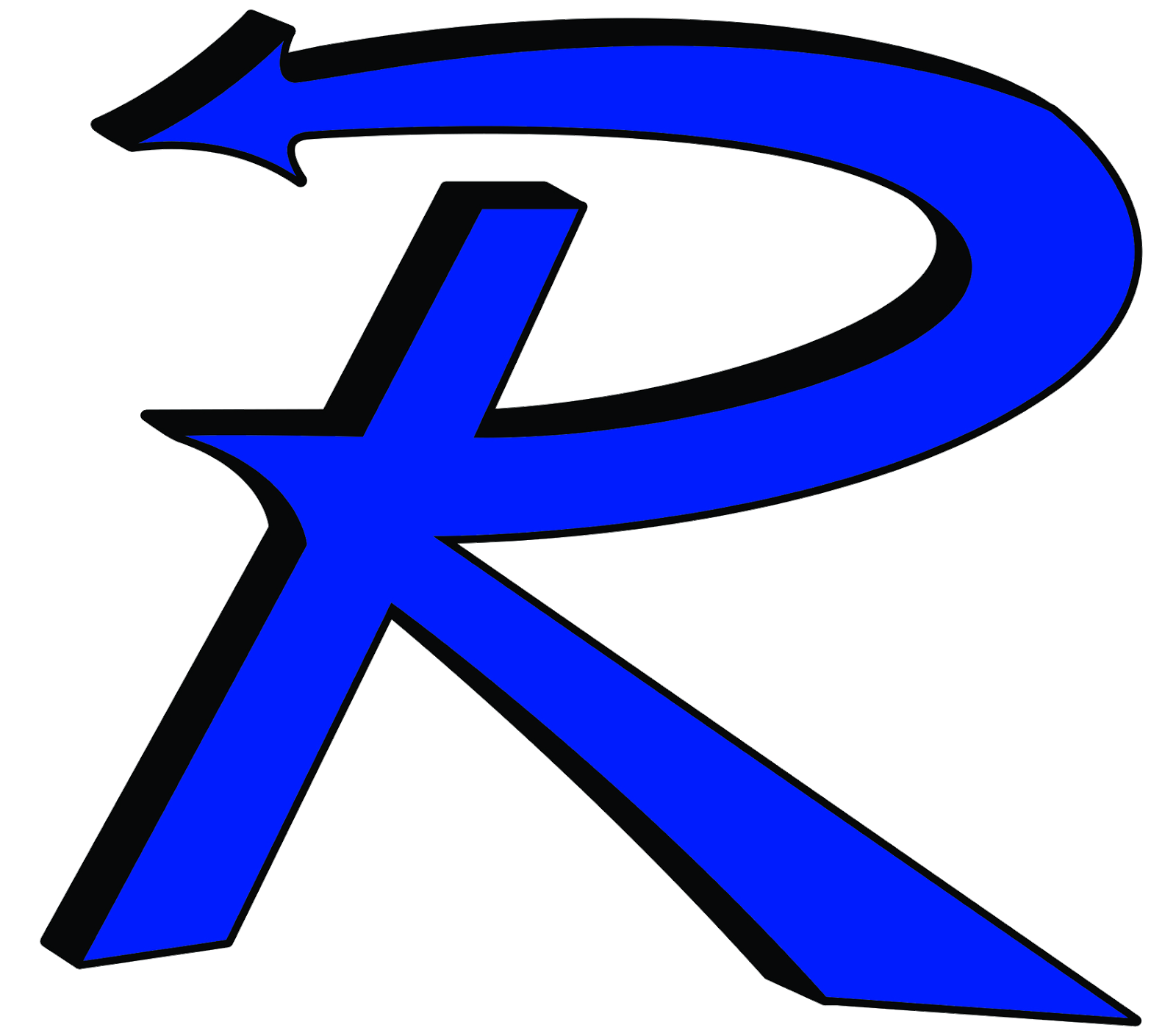## Jan Aldridge

 Welcome to Mathematics (GTHNRS Algebra II, Math Models,CP Math, and Algebra II) Jan Aldridge (903)671-3421Click here to send a message.

Daily Schedule

 1st Period 8:00-8:50 Algebra II 2nd Period 8:53-9:42 GTHNRS Algebra II 3rd Period 9:46-10:35 CP Mathematics 4th Period 10:39-11:28 Algebra II 5th Period 11:32-12:21 CP Mathematics 6th Period 12:55-1:44 Conference 7th Period 1:48-2:37 Math Models 8th Period 2:41-3:30

Syllabus

Student Assignments

GTHNRS GEOMETRY SUPPLY LIST

• 2 Red Pens
• Several #2 Pencils AND Erasers
• Notebook Paper
• Pronged 2 pocket Folder
• Graphing Paper ( doesn’t matter what type)
• Straight edge (protractor that measures)
• Compass
• Scissors
• Graphing Calculator ( You may rent or buy)

MATH MODELS & CP MATH SUPPLY LIST

• Notebook Paper
• Pronged 2 pocket folder
• Graph paper (it doesn’t matter what type)
• Graphing Calculator ( you may rent or buy)
• 2 Red Pens

• ALGEBRA II SUPPLY LIST
• Graphing Paper ( it doesn’t matter what type)
• 2 Red Pens
• Several #2 Pencils AND Erasers
• Notebook Paper (Lots)
• Pronged 2 pocket Folder
• Graphing Calculator (You may rent or buy)
• Composition Notebook (100 Sheets --Sewn not glued)
• Elmers white regular glue

• GTHNRS ALGEBRA II SUPPLY LIST

• Graphing Paper (it doesn’t matter what type)
• 2 Red Pens
• Several #2 Pencils AND Erasers
• Notebook paper (Lots)
• Graphing Calculator (You may rent or buy)

GTHNRS Geometry Course Description

This is a one year course to develop and practice problem-solving skills using inductive and deductive reasoning.  Students are guided through all the conceptual and working levels of the process using geometry.   It uses two and three-dimensional geometric shapes (Points, lines, planes, triangles, polygons, circles, and solids) and examines their properties, measurements, and mutual relations in space. Geometric proofs are used as a vehicle to systematically develop these problem solving skills by relating geometric shapes.

Algebra II Course Description

This course is a continuation of algebraic and geometric concepts developed in Algebra I and Geometry.  Students will review solving equations, inequalities, and graphing functions.  The students will continue their foundation of functions, use symbol and manipulations to simplify and solve, connect algebra and geometry, work different methods for solving systems of equations, matrices, quadratic, square root, rational, exponential and logarithmic functions.  Students will continue to build on this foundation as they expand their understanding through other mathematical experiences.

Math Models Course Description

Students use algebraic, graphical, and geometric reasoning to recognize patterns and structure, to model information, and to solve problems from various disciplines.  Students use mathematical methods to model and solve real-life applied problems involving money, data, chance, patterns, music, design, and science.  Students use mathematical models from algebra, geometry, probability, and statistics and connections among these to solve problems from a wide variety of advanced applications in both mathematical and nonmathematical situations.  Students use a variety of representations (concrete, pictorial, numerical, symbolic, graphical, and verbal), tools, and technology (including, but not limited to, calculators with graphing capabilities, data collection devices, and computers) to link modelling techniques and purely mathematical concepts and to solve applied problems.

 GT Honors Algebra 2 Course Description GT Honors Algebra 2 allows students to build on the algebraic skills of Algebra 1. It shows a connection between Algebra and Geometry and illustrates how the tools of one can be used to solve problems in the other. It also extends knowledge to include more extensive data analysis and problem solving which is necessary to be successful in the higher mathematics classes. Prerequisites: Algebra 1, Geometry, and teacher recommendation.   College-Prep Mathematics Course Description the purpose of this course is to provide students with a solid foundation in Algebra.  this course is designed to prepare students for their next mathematics course or for the courses that are nonmathematical but require an understanding of algebraic fundamentals.  Topics that will be covered include:  equations, inequalities, problem solving, exponents, polynomials, graphing, factoring, rational expressions, absolute value, rational exponents, radicals, and complex numbers.  The goal for this course is for the student to be prepared for the next step in their educational/creer choice.